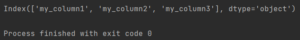Pandas How To Uncategorized How to get column names

# How to get column names

Here’s how to get column names in Pandas.To get the column names of your dataframe in Pandas you only need to use the columns function.

## How to get column names

```import pandas as pd

df1 = pd.DataFrame({"my_column1": ["something", "somethingelse"],
"my_column2": ['foo', 'bar'],
"my_column3": ['some', 'data']})

print(df1.columns)

```

Output:

```Index(['my_column1', 'my_column2', 'my_column3'], dtype='object')

```

Pandas lists all the columns present in my dataframe.

## How to get a list of column names

If such an output is problematic for you or you need it as a data source, use the to_list method. Using the to_list function, Pandas will return the column names as a list.

```import pandas as pd

df1 = pd.DataFrame({"my_column1": ["something", "somethingelse"],
"my_column2": ['foo', 'bar'],
"my_column3": ['some', 'data']})

print(df1.columns.values.tolist())

```

Output:

```['my_column1', 'my_column2', 'my_column3']
```

## How to get the name of a specific column

Using the columns function you can also easily get the name of a specific column. For example, I will write code that displays the first column. I will use index 0.

```import pandas as pd

df1 = pd.DataFrame({"my_column1": ["something", "somethingelse"],
"my_column2": ['foo', 'bar'],
"my_column3": ['some', 'data']})

print(df1.columns)

```

Output:

```my_column1
```

You learned how to get the column names and how to get the column name by index. You can also save output as a list.

## How to join dataframe with seriesHow to join dataframe with series

You can join a dataframe with a series in pandas using the merge method or the concat function. The default behavior is to align the series index with the dataframe

## How to solve RuntimeError in PandasHow to solve RuntimeError in Pandas

A RuntimeError in Pandas typically occurs when there is an error in your code related to the execution of the program.

## How to cast column to int in PandasHow to cast column to int in Pandas

To cast a column to integer in Pandas, you can use the astype() method. Here’s an example: